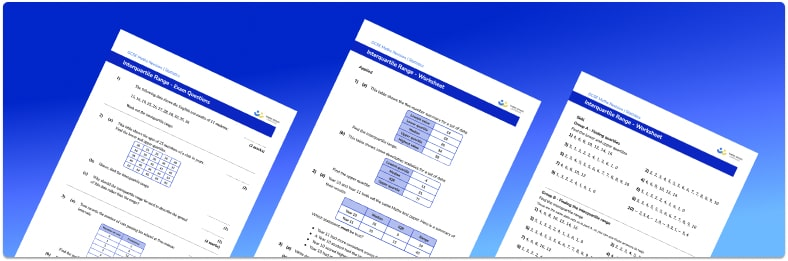# Interquartile Range Worksheet• Section 1 of the interquartile range worksheet contains 24 skills-based interquartile range questions, in 3 groups to support differentiation
• Section 2 contains 3 applied interquartile range questions with a mix of word problems and deeper problem solving questions
• Section 3 contains 3 foundation and higher level GCSE exam style interquartile range questions
• An answer key and a mark scheme for all interquartile range questions are provided
• Questions follow variation theory with plenty of opportunities for students to work independently at their own level
• All questions created by fully qualified expert secondary maths teachers

Suitable for GCSE maths revision for AQA, OCR and Edexcel exam boards

• This field is for validation purposes and should be left unchanged.

You can unsubscribe at any time (each email we send will contain an easy way to unsubscribe). To find out more about how we use your data, see our privacy policy.

### Interquartile range at a glance

The interquartile range (or IQR) is a measure of spread or variability, and is used alongside the median as a measure of central tendency. To calculate the interquartile range for a set of data, we first find the lower quartile (the data item one quarter or 25% of the way through the data set) and the upper quartile (the data item three quarters or 75% of the way through the data set). We then subtract the lower quartile from the upper quartile to get the interquartile range.

A box and whisker plot is a graph showing the lower quartile (first quartile), median (second quartile), and upper quartile (third quartile), along with the minimum and maximum data points. The interquartile range of the data can be found from a box plot by identifying the lower and upper quartiles and proceeding as above.

The main advantage of using the median and the interquartile range is that they are not affected by extreme values. They are therefore appropriate for use with a skewed data set, or one with outliers.

Looking forward, students can then progress to additional Statistics worksheets, for example the box and whisker plot worksheet, the mean, median and mode worksheet, or the cumulative frequency worksheet.

For more teaching and learning support on Statistics our GCSE maths lessons provide step by step support for all GCSE maths concepts.

## Do you have KS4 students who need more focused attention to succeed at GCSE?There will be students in your class who require individual attention to help them succeed in their maths GCSEs. In a class of 30, it’s not always easy to provide.

Help your students feel confident with exam-style questions and the strategies they’ll need to answer them correctly with our dedicated GCSE maths revision programme.

Lessons are selected to provide support where each student needs it most, and specially-trained GCSE maths tutors adapt the pitch and pace of each lesson. This ensures a personalised revision programme that raises grades and boosts confidence.

Find out more